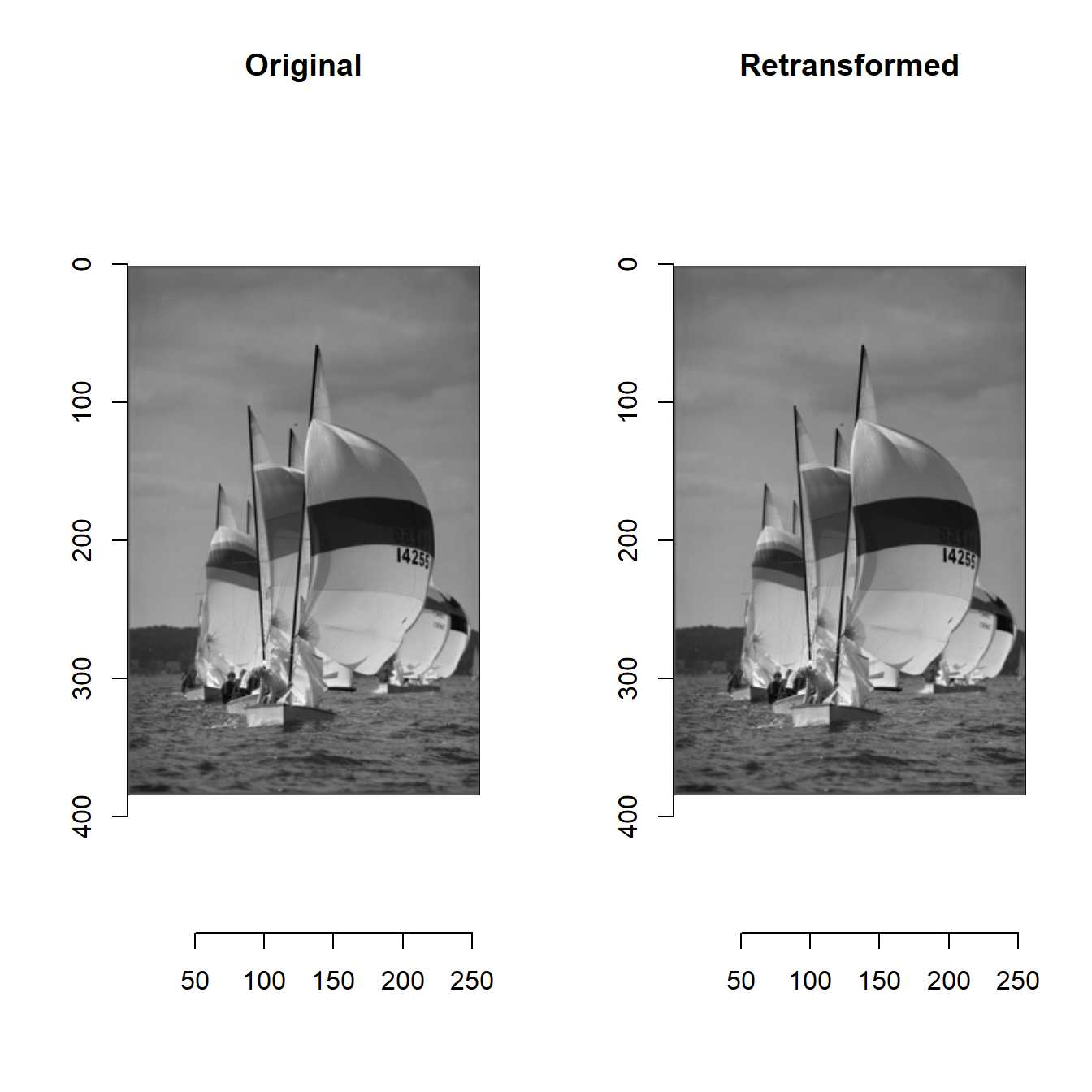Fast Discrete Cosine Transformation

Discrete cosine transform (DCT) is an important tool in the field of image processing.

DCT transforms a signal into the frequencies.

Many of the signal informations are transformed into low frequencies.

Thus, DCT is suitable for frequency filter.

This is the distionction of DCT.

I implemented two dimensional discrete cosine transform (DCT2D) and two dimensional inverse discrete cosine transform (IDCT2D).

You can confirm it by the code below.

library(devtools)
install_github("ShotaOchi/imagerExtra")
library(imagerExtra)
layout(matrix(1:2, 1, 2))
g <- grayscale(boats) %>% plot(main = "Original")
gg <- DCT2D(g) %>% IDCT2D() %>% plot(main = "Retransformed")mean((g - gg)^2)
 2.389584e-32